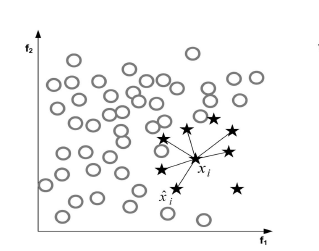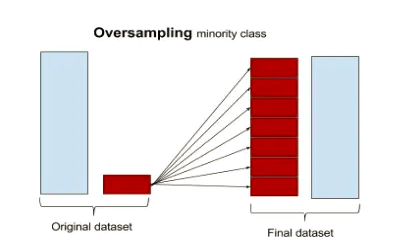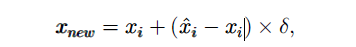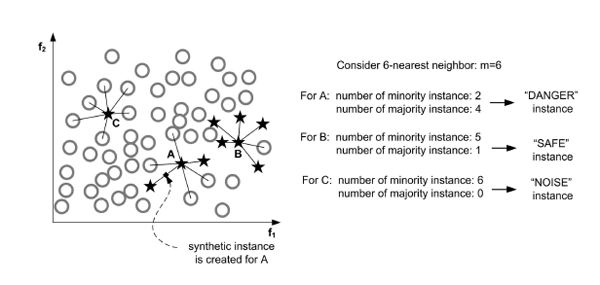# Python中怎么处理不平衡数据集

1. 什么是数据不平衡

•  信用卡欺诈数据：99%都是正常的数据， 1%是欺诈数据

•  贷款逾期数据

•  欠采样

•  过采样

•  综合采样

•  模型集成

•  调整类别权重或者样本权重

2. 数据不平衡处理方法

imbalanced-learn库提供了许多不平衡数据处理的方法，本文的例子都以imbalanced-learn库来实现。

`pip install -U imbalanced-learn`

https://github.com/scikit-learn-contrib/imbalanced-learn

`import pandas as pd  train_data = './data/train.csv'  test_data = './data/test.csv'  train_df = pd.read_csv(train_data)  test_df = pd.read_csv(test_data)  print(train_df.groupby(['label']).size())  # label为是否违约， 1为违约， 0为非违约  #     label  # 0    37243  # 1     2757`2.1 欠采样•  一种是overlapping的数据，就是多余的数据

•  一种是干扰的数据，干扰minority的分布`from imblearn.under_sampling import TomekLinks  X_train = train_df.drop(['id', 'type'], axis=1)  y = train_df['label']  tl = TomekLinks()  X_us, y_us = tl.fit_sample(X_train, y)  print(X_us.groupby(['label']).size())  # label  # 0    36069  # 1     2757`

•  聚类

这类方法通过多个聚类，把原始样本划分成多个聚类簇，然后用每个聚类簇的中心来代替这个聚类簇的特性，完成采样的目的。可知，这种采样的样本不是来自原始样本集，而是聚类生成              的。

`from imblearn.under_sampling import ClusterCentroids       cc = ClusterCentroids(random_state=42)      X_res, y_res = cc.fit_resample(X_train, y)      X_res.groupby(['label']).size()      # label      # 0    2757      # 1    2757`

im-balance提供的欠采样的方法如下：

•  Random majority under-sampling with replacement

•  Extraction of majority-minority Tomek links

•  Under-sampling with Cluster Centroids

•  NearMiss-(1 & 2 & 3)

•  Condensed Nearest Neighbour

•  One-Sided Selection

•  Neighboorhood Cleaning Rule

•  Edited Nearest Neighbours

•  Instance Hardness Threshold

•  Repeated Edited Nearest Neighbours

•  AllKNN

2.2 过采样SMOTE基于minority样本相似的特征空间构造新的人工样本。步骤如下：

•  选择一个minority样本，计算其KNN邻居

•  在K个邻居中，随机选择一个近邻

•  修改某一个特征,偏移一定的大小：偏移的大小为该minority样本与该近邻差距乘以一个小的随机比率(0, 1)， 就此生成新样本`from imblearn.over_sampling import SMOTE  smote = SMOTE(k_neighbors=5, random_state=42)  X_res, y_res = smote.fit_resample(X_train, y)  X_res.groupby(['label']).size()  # label  # 0    37243  # 1    37243``from imblearn.over_sampling import BorderlineSMOTE  bsmote = BorderlineSMOTE(k_neighbors=5, random_state=42)  X_res, y_res = bsmote.fit_resample(X_train, y)  X_res.groupby(['label']).size()  # label  # 0    37243  # 1    37243`

•  先确定要生成样本的数量 beta为[0, 1]•  对每个每个minortiy样本，确定有它生成样本的比例。先找出K最近邻，计算K最近邻中属于majority的样本比例（即分子），Z是归一化因子，保证所有的minortiry的比例和为1，可以认为是所有分子的和。•  计算每个minortiy生成新样本的数量•  按照SMOTE方式生成样本

`from imblearn.over_sampling import ADASYN   adasyn = ADASYN(n_neighbors=5, random_state=42)  X_res, y_res = adasyn.fit_resample(X_train, y)  X_res.groupby(['label']).size()  # label  # 0    37243  # 1    36690`

im-balance提供的过采样的方法如下（包括SMOTE算法的变种）：

•  Random minority over-sampling with replacement

•  SMOTE - Synthetic Minority Over-sampling Technique

•  SMOTENC - SMOTE for Nominal Continuous

•  bSMOTE(1 & 2) - Borderline SMOTE of types 1 and 2

•  SVM SMOTE - Support Vectors SMOTE

•  KMeans-SMOTE

•  ROSE - Random OverSampling Examples

2.3 综合采样

`from imblearn.combine import SMOTETomek  smote_tomek = SMOTETomek(random_state=0)  X_res, y_res = smote_tomek.fit_sample(X_train, y)  X_res.groupby(['label']).size()  # label  # 0    36260  # 1    36260`

2.4 模型集成

`from imblearn.ensemble import BalancedRandomForestClassifier  from sklearn.datasets import make_classification  X, y = make_classification(n_samples=1000, n_classes=3,                             n_informative=4, weights=[0.2, 0.3, 0.5],                             random_state=0)  clf = BalancedRandomForestClassifier(max_depth=2, random_state=0)  clf.fit(X, y)    print(clf.feature_importances_)    print(clf.predict([[0, 0, 0, 0, 0, 0, 0, 0, 0, 0,                      0, 0, 0, 0, 0, 0, 0, 0, 0, 0]]))`

im-balance提供的模型集成的方法如下

•  Easy Ensemble classifier

•  Balanced Random Forest

•  Balanced Bagging

•  RUSBoost

2.5 调整类别权重或者样本权重

`import lightgbm as lgb  clf = lgb.LGBMRegressor(num_leaves=31,                           min_child_samples= np.random.randint(20,25),                          max_depth=25,                          learning_rate=0.1,                           class_weight={0:1, 1:10},                          n_estimators=500,                           n_jobs=30)`